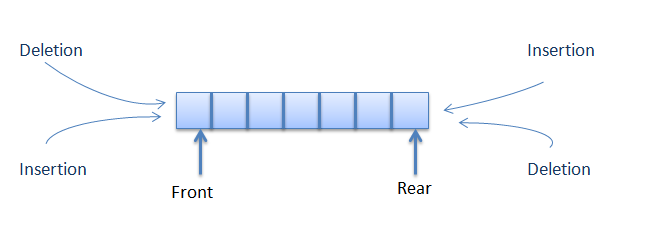Data Structure

# Double-Ended Queue

A double-ended queue is an abstract data type similar to an simple queue, it allows you to insert and delete from both sides means items can be added or deleted from the front or rear end.# Algorithm for Insertion at rear end

```Step -1: [Check for overflow]

if(rear==MAX)

Print("Queue is Overflow”);

return;

Step-2: [Insert element]

else

rear=rear+1;

q[rear]=no;

[Set rear and front pointer]

if rear=0

rear=1;

if front=0

front=1;

Step-3: return

```

# Implementation of Insertion at rear end

```void add_item_rear()

{

int num;

printf("\n Enter Item to insert : ");

scanf("%d",&num);

if(rear==MAX)

{

printf("\n Queue is Overflow");

return;

}

else

{

rear++;

q[rear]=num;

if(rear==0)

rear=1;

if(front==0)

front=1;

}

}

```

# Algorithm for Insertion at font end

```Step-1 : [Check for the front position]

if(front<=1)

Print (“Cannot add item at front end”);

return;

Step-2  : [Insert at front]

else

front=front-1;

q[front]=no;

Step-3  : Return

```

# Implementation of Insertion at font end

```void add_item_front()

{

int num;

printf("\n Enter item to insert:");

scanf("%d",&num);

if(front<=1)

{

printf("\n Cannot add item at front end");

return;

}

else

{

front--;

q[front]=num;

}

}

```

# Algorithm for Deletion from front end

```Step-1 [ Check for front pointer]

if front=0

print(" Queue is Underflow”);

return;

Step-2 [Perform deletion]

else

no=q[front];

print(“Deleted element is”,no);

[Set front and rear pointer]

if front=rear

front=0;

rear=0;

else

front=front+1;

Step-3 : Return

```

# Implementation of Deletion from front end

```void delete_item_front()

{

int num;

if(front==0)

{

printf("\n Queue is Underflow\n");

return;

}

else

{

num=q[front];

printf("\n Deleted item is %d\n",num);

if(front==rear)

{

front=0;

rear=0;

}

else

{

front++;

}

}

}

```

# Algorithm for Deletion from rear end

```Step-1 : [Check for the rear pointer]

if rear=0

print(“Cannot delete value at rear end”);

return;

Step-2: [ perform deletion]

else

no=q[rear];

[Check for the front and rear pointer]

if front= rear

front=0;

rear=0;

else

rear=rear-1;

print(“Deleted element is”,no);

Step-3 : Return

```

# Implementation of Deletion from rear end

```void delete_item_rear()

{

int num;

if(rear==0)

{

printf("\n Cannot delete item at rear end\n");

return;

}

else

{

num=q[rear];

if(front==rear)

{

front=0;

rear=0;

}

else

{

rear--;

printf("\n Deleted item is %d\n",num);

}

}

}

```